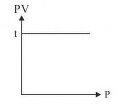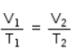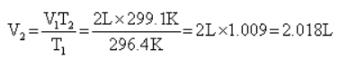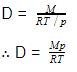Courses

# Test: Gas Laws

## 15 Questions MCQ Test Chemistry Class 11 | Test: Gas Laws

Description
This mock test of Test: Gas Laws for JEE helps you for every JEE entrance exam. This contains 15 Multiple Choice Questions for JEE Test: Gas Laws (mcq) to study with solutions a complete question bank. The solved questions answers in this Test: Gas Laws quiz give you a good mix of easy questions and tough questions. JEE students definitely take this Test: Gas Laws exercise for a better result in the exam. You can find other Test: Gas Laws extra questions, long questions & short questions for JEE on EduRev as well by searching above.
QUESTION: 1

### The slope of plot between pV and p at constant temperature is:

Solution:

Slope of the plot between P and PV at constant temperature is zero. A plot of P v/s PV at constant temperature for a fixed mass of gas is a straight line parallel to the pressure axis.QUESTION: 2

Solution:
QUESTION: 3

### At constant temperature, the pressure of a fixed amount of gas varies inversely with its volume, is

Solution:

The correct answer is option A.
Boyle's law:
In 1662 Robert Boyle studied the relationship between volume and pressure of a gas of fixed amount at constant temperature. He observed that volume of a given mass of a gas is inversely proportional to its pressure at a constant temperature.

QUESTION: 4

Dalton’s law of partial pressure states that

Solution:

The correct answer is option B
Dalton's law (also called Dalton's law of partial pressures) states that in a mixture of non-reacting gases, the total pressure exerted is equal to the sum of the partial pressures of the individual gases. This empirical law was observed by John Dalton in 1801 and published in 1802.

QUESTION: 5

At constant volume, pressure of a fixed amount of a gas varies directly with the temperature, is

Solution:

Gay Lussac’s law states that the pressure of a fixed mass of a gas at constant volume is directly proportional to the temperature on Kelvin scale.

QUESTION: 6

The vapour pressure of water at 300 K in a closed container is 0.4 atm. If the volume of the container is doubled, its vapour pressure at 300 K will be

Solution:

The vapour pressure of water will remain the same as the temperature is unchanged. So, the answer is c) 0.4 atm

QUESTION: 7

The value of universal gas constant R depends on:

Solution:

We have

R = PV/T

= 8.314JK−1mol−1

= 8.314x107ergK−1mol−1

= 2calK−11mol−1

= 0.0821litreatmK−1mol−1

Hence R depends on units of volume and pressure.

QUESTION: 8

Equal volumes of all gases under the same conditions of temperature and pressure contain equal number of molecules, is

Solution:

Avogadro's law states that, "equal volumes of all gases, at the same temperature and pressure, have the same number of molecules."

QUESTION: 9

Use of hot air balloons is an application of:

Solution:

The relationship between the temperature and volume of a gas, which is known as Charles' law, provides an explanation of how hot-air balloons work.

QUESTION: 10

A vessel contains 0.16g of methane and 0.28g of nitrogen. The ratio of partial pressures of methane to nitrogen in the mixture is about:

Solution:

We know, Partial pressure of a gas = mole fraction of that gas x Total pressure of mixture of the gases.

Using this formula and using the information given in this question, we get
ratio of partial pressures of methane to nitrogen in the mixture as: 1:1

QUESTION: 11

A balloon seller filled a balloon with 2 L air when the temperature was 23.4° C. He sold the balloon at the time when temperature was recorded to 26.1°C. Volume of the balloon at this temperature is

Solution:

From Charles law we conclude thatQUESTION: 12

Graph for Boyle's law is called

Solution:

For Boyle’s law, temperature is constant. So the graph is called as isotherm.

QUESTION: 13

One mole of N2O4 is kept in a close container at 300K and 1 atm pressure. When it is heated to 600 K 20% of it decomposes to NO2 (g). Calculate the resultant pressure exerted on the walls of the container

Solution:

N2O⇔ 2NO2
1mole 0 Initial moles
-20% of 1=0.2  +2(20% of 1)=0.40  At equilibrium
1-0.2= 0.8  0.4  Moles at equilibrium
Total moles at equilibrium = 0.8+0.4 = 1.2 moles
1 mole vapour pressure = 1 atm at 300 K
Applying PV = nRT
1xV = 1x R x 300 .... (1)
When n=1.2 moles, T = 600 K
P x V = 1.2 x R x 600 .... (2)
Dividing (2) by (1),
PxV/(1xV) = (1.2 x R x 600)/ (1 x R x 300)
Therefore, P= 2.4 atm
Hence, resultant pressure of mixture is 2.4 atm

QUESTION: 14

The density of the gas is equal to:

Solution:

The correct answer is Option C.
For ideal gas
pV=RT
V=RT/p
Density is given by
D=M/V
Where M is mass and V is volume
Putting the value of VQUESTION: 15

For a certain reaction PV = 2 dm3 atm and volume is 4 dm3 then corresponding pressure is

Solution:

P1V1=P2V2
2=P2×4
P2=0.5